# How Aggregations Work

SQL COUNT is the most common type of Aggregation in SQL, it counts the number of rows in a column or table. COUNT(*) tells SQL to count the number of rows of the whole table. COUNT(some column) tells SQL to count the number of non-null rows in that column.

``````SELECT COUNT(*)
``````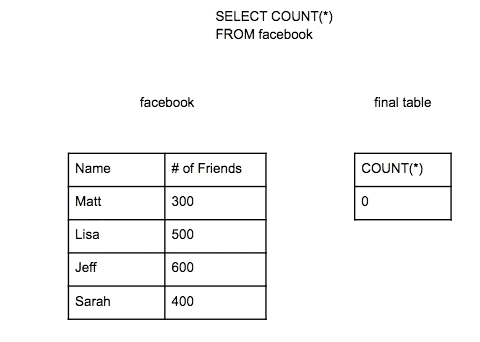SQL goes through all the rows of the table and creates a table with total count of rows.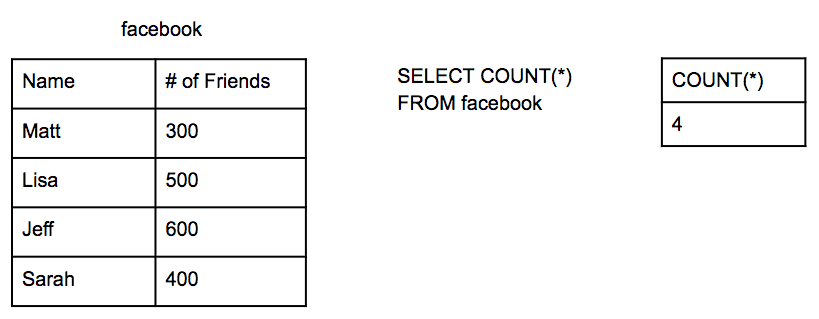With more complex data, SQL can answer more complex questions such as how many rows are there per state.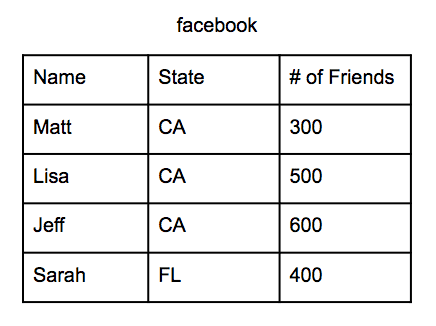The COUNT aggregation can answer this, but SQL needs to be told what to group by in the query. SQL also needs the column it is grouping by in the SELECT statement so that the final table can show the data in its proper groups.

``````SELECT State, COUNT(*)
GROUP BY State
``````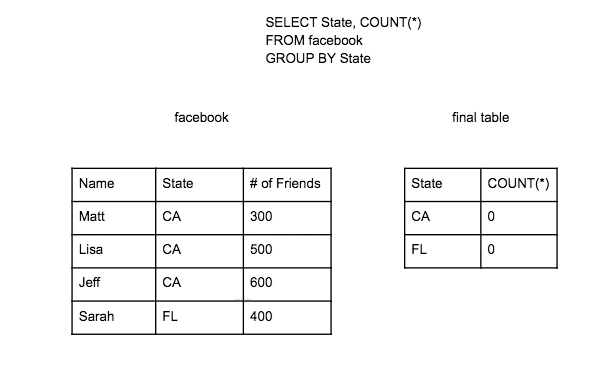First SQL will group together data by the column or columns listed in the group by statement in this case state. Then SQL will perform the same COUNT operation as before but check which group to assign the count to.

The data and question can be even more complicated, in this example there is a city column in the table as well. The question could be how many rows are there for each State and City.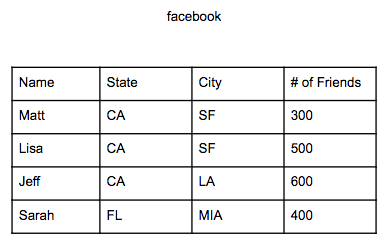The COUNT aggregation will get us our answer as long as it is grouped by state and city. Remember SQL needs what we are grouping by in the SELECT statement as well so that these groups will show in the final table.

``````SELECT State, City, COUNT(*)
GROUP BY State, City
``````## SUM and AVERAGE Aggregation

After COUNT, for all other types of aggregation will need you to define the column you are aggregating.

Wrong:

``````SELECT SUM(*)
``````

Right:

``````SELECT SUM(# of friends)
``````

How would it SUM all the columns in the facebook table? The name column has text and cannot summed. Also there are multiple columns so we cannot SUM the row as a whole like the way we could COUNT the row as a whole. SQL needs to know which column the aggregation should be on, in this case the only numeric column is # of friends.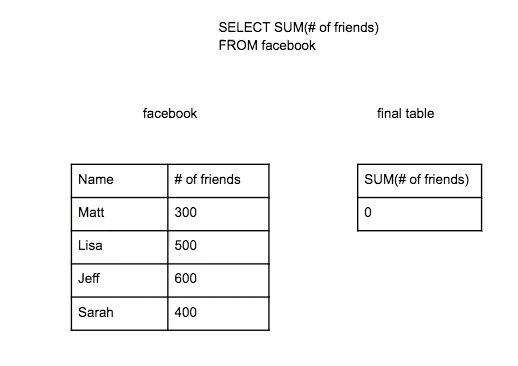The Average aggregation operates similarly to SUM. The data will be more complex for this example.

``````SELECT State, AVG(# of friends)
GROUP BY State
``````To get an average SQL needs the sum of each group and then divide it by the count of rows in each group.

## NULLs

There are a few scenarios to be aware of when aggregating data that may make you misinterpret the results of your query.

Some cells will not have a value in it. This type of cell is considered null. Null is different than 0 or space “ ”.

The COUNT(*) aggregation will count all rows including Null values. However the COUNT(some column) will count all rows without Null values. Since other aggregations require you specify a column they will exclude Nulls in their calculations.

COUNT(*) counts Nulls: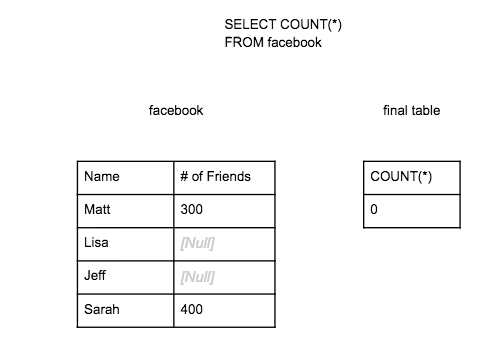COUNT(# of friends) does not count Nulls:Written by: Matt David
Reviewed by:

Our Web Books